# 1 Prerequisites

You need to have installed R and a suitable editor such as RStudio or VSCode with R plugin. See the package’s README for instructions on installing the {simodels} package.

library(simodels)
library(dplyr)
#>
#> Attaching package: 'dplyr'
#> The following objects are masked from 'package:stats':
#>
#>     filter, lag
#> The following objects are masked from 'package:base':
#>
#>     intersect, setdiff, setequal, union
library(ggplot2)

# 2 Input data

This tutorial builds on a reproducible guide to SIMs in R (Dennett 2018). We start by importing open access data representing movement between zones in Australia (thanks to Adam Dennett for making the files accessible):

u1 = "https://github.com/Robinlovelace/simodels/releases/download/0.0.1/zones_aus.geojson"
u2 = "https://www.dropbox.com/s/wi3zxlq5pff1yda/AusMig2011.csv?raw=1"
od_aus = read.csv(u2)

Let’s take a quick look at and ‘minimize’ these input datasets before modelling them with SIMs:

dim(zones_aus)
#>  15  7
names(zones_aus)
#>  "GCCSA_CODE" "GCC_CODE16" "GCCSA_NAME" "STATE_CODE" "STATE_NAME"
#>  "AREA_SQKM"  "geometry"
key_zone_names = c("GCCSA_CODE", "GCCSA_NAME", "AREA_SQKM")
zones = zones_aus[key_zone_names]
#> Simple feature collection with 2 features and 3 fields
#> Geometry type: MULTIPOLYGON
#> Dimension:     XY
#> Bounding box:  xmin: 140.9993 ymin: -37.50503 xmax: 153.6298 ymax: -28.16441
#> Geodetic CRS:  GDA94
#> # A tibble: 2 × 4
#>   GCCSA_CODE GCCSA_NAME     AREA_SQKM                                   geometry
#>   <chr>      <chr>              <dbl>                         <MULTIPOLYGON [°]>
#> 1 1RNSW      Rest of NSW      788443. (((153.008 -28.34026, 153.11 -28.35709, 1…
#> 2 1GSYD      Greater Sydney    12368. (((151.5961 -33.16168, 151.6305 -33.17709…
dim(od_aus)
#>  225  13
names(od_aus)
#>   "Origin"          "Orig_code"       "Destination"     "Dest_code"
#>   "Flow"            "vi1_origpop"     "wj1_destpop"     "vi2_origunemp"
#>   "wj2_destunemp"   "vi3_origmedinc"  "wj3_destmedinc"  "vi4_origpctrent"
#>  "wj4_destpctrent"
key_od_names = c("Orig_code", "Dest_code", "Flow")
od = od_aus[key_od_names]
#>   Orig_code Dest_code    Flow
#> 1     1GSYD     1GSYD 3395015
#> 2     1GSYD     1RNSW   91031

The results printed above show that:

• zones_aus represents the 15 regions of Australia, with columns containing zone identifiers (IDs), primarily GCCSA_CODE, and the area of each zone (AREA_SQKM). The minimal zones object contains only the region code, name, and area.

• od_aus contains 225 rows of data representing the movement of people between the zones in aus. Note that 255 is 15 squared, meaning that this OD dataset contains the complete combination of migration flows, starting with zone 1GSYD to 1GSYD. These codes are present in the GCCSA_CODE column in the aus object. The first row of the OD dataset represents ‘intra-zonal’ migrations in Greater Sydney, presumably counting the number of people who move house from somewhere in the region to another home in the region. There are 13 columns in this dataset, the most important of which are Orig_code, Dest_code, and Flow, which we have captured in the minimal od dataset.

Note: a useful convention with ‘long form’ OD datasets is for the first two columns to contain zone IDs that correspond to values in the first column of the zone dataset. The R package {od}, on which {simodels} builds, assumes that inputs to its functions are in this form.

It is a good idea to verify that the origin and destination codes in the od dataset match the zone codes in zones:

summary(od[] %in% zones[])
#>    Mode    TRUE
#> logical     225
summary(od[] %in% zones[])
#>    Mode    TRUE
#> logical     225

It is clear from the above that we have ‘clean’ input datasets, let’s begin with the modelling!

# 3 Preparing a SIM

Key to the workings of the {simodels} package is the conversion of geographic objects representing origins and destinations into an OD dataset. In this case, we already have an OD dataset, so this step is less relevant. However, we will take this step in any case because many SIMs start without such a comprehensive OD dataset as we have in this case.

Prepare the OD dataset as follows:

od_sim = si_to_od(origins = zones, destinations = zones)
#> Maximum distance is > 100km. The 'cheap' measure is inaccurate over such
#> large distances, you'd likely be better using a different 'measure'.
names(od_sim)
#>  "O"                      "D"                      "distance_euclidean"
#>  "origin_GCCSA_NAME"      "origin_AREA_SQKM"       "destination_GCCSA_NAME"
#>  "destination_AREA_SQKM"  "geometry"

Note that the output has duplicate columns: si_to_od() joins data from the origin and destination objects into the resulting OD object.

# 4 An unconstrained SIM

A simplistic SIM - in this case an inverse power distance decay function (negative exponential is another commonly used decay function) - can be created just based on the distance between points:

si_power = function(d, beta) (d / 1000)^beta
od_calculated = si_calculate(
od_sim,
fun = si_power,
d = distance_euclidean,
beta = -0.8
)
plot(od_calculated["interaction"], logz = TRUE)
#> Warning in classInt::classIntervals(v0, min(nbreaks, n.unq), breaks, warnSmallN
#> = FALSE): var has infinite values, omitted in finding classes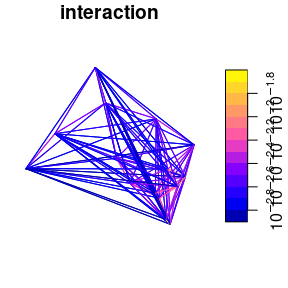This approach, ignoring all variables at the level of trip origins and destinations, results in flow estimates with no units. Before learning how to run constrained SIMs, let’s scale the result by the total flow and see how far we are from reality, just focussing on the interzonal OD pairs:

od_interzonal = od %>%
filter(Orig_code != Dest_code)
od_calculated_interzonal = od_calculated %>%
filter(O != D)
scale_factor = sum(od_interzonal$Flow) / sum(od_calculated_interzonal$interaction)
od_calculated_interzonal = od_calculated_interzonal %>%
mutate(interaction_scaled = interaction * scale_factor)
od_joined = inner_join(
od_calculated_interzonal,
od %>% rename(O = Orig_code, D = Dest_code)
)
#> Joining, by = c("O", "D")
od_joined %>%
ggplot() +
geom_point(aes(Flow, interaction_scaled))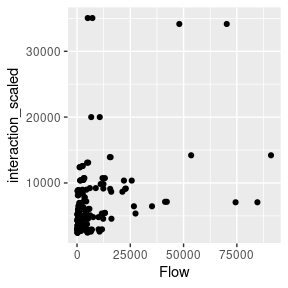cor(od_joined$Flow, od_joined$interaction_scaled)^2
#>  0.1677679

The results show that a simple unconstrained model, without any parameter fitting, can explain less than 20% of the variability in flows. We can do better!

od_joined %>%
mutate(decay = distance_euclidean^-0.8) %>%
mutate(decay = decay * (sum(Flow) / sum(decay))) %>%
ggplot() +
geom_point(aes(distance_euclidean, Flow)) +
geom_line(aes(distance_euclidean, decay), colour = "red")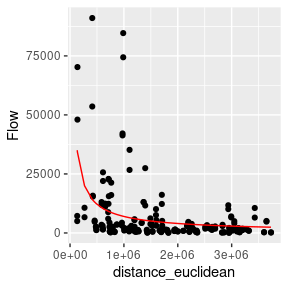# 5 A production constrained SIM

The first logical way to improve model fit is to run a production constrained model. To do that, we’ll first calculate the total number of people leaving each zone and then use the constraint_production argument:

od_originating = od_joined %>%
group_by(O) %>%
mutate(originating_per_zone = sum(Flow)) %>%
ungroup()
od_constrained_p = si_calculate(
od_originating,
fun = si_power,
d = distance_euclidean,
beta = -0.8,
constraint_production = originating_per_zone
)
od_constrained_p %>%
ggplot() +
geom_point(aes(Flow, interaction))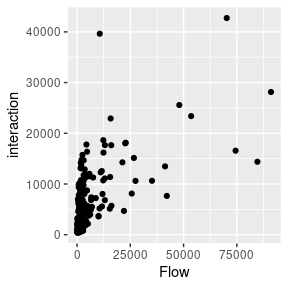cor(od_constrained_p$Flow, od_constrained_p$interaction)^2
#>  0.3891644

Progress! We have more than doubled the predictive ability of our model by using a ‘production constrained’ SIM, as defined mathematically in the simodels-first-principles vignette.

# 6 Training a SIM

An advantage of the flow data used in this example is that we already know the interaction. (This raises the question of why a SIM is needed, answer: to test our models and demonstrate the techniques.)

We can do this using the nls() function as follows:

library(minpack.lm)
f = Flow ~ a * (distance_euclidean)^b
m = nlsLM(
formula = f,
data = od_originating,
)
#> Warning in nlsLM(formula = f, data = od_originating, ): No starting values specified for some parameters.
#> Initializing 'a', 'b' to '1.'.
#> Consider specifying 'start' or using a selfStart model
#> Warning in nls.lm(par = start, fn = FCT, jac = jac, control = control, lower = lower, : lmdif: info = -1. Number of iterations has reached maxiter' == 50.
m
#> Nonlinear regression model
#>   model: Flow ~ a * (distance_euclidean)^b
#>    data: od_originating
#>          a          b
#>  2.182e+07 -5.801e-01
#>  residual sum-of-squares: 3.089e+10
#>
#> Number of iterations till stop: 50
#> Achieved convergence tolerance: 1.49e-08
#> Reason stopped: Number of iterations has reached maxiter' == 50.
# Nonlinear regression model
#   model: Flow ~ a * (distance_euclidean)^b
#    data: od_originating
#          a          b
#  2.182e+07 -5.801e-01 
od_joined %>%
mutate(decay = distance_euclidean^-5.801e-01) %>%
mutate(decay = decay * 2.182e+07) %>%
ggplot() +
geom_point(aes(distance_euclidean, Flow)) +
geom_line(aes(distance_euclidean, decay), colour = "red")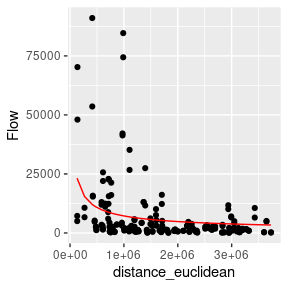od_pred = si_predict(od_originating, model = m)
cor(od_pred$Flow, od_pred$interaction)^2
#>  0.1746446
od_pred_const = si_predict(od_originating, model = m,
constraint_production = originating_per_zone)
cor(od_pred_const$Flow, od_pred_const$interaction)^2
#>  0.338483
library(tmap)
ttm()
#> tmap mode set to interactive viewing
tm_shape(od_pred_const) +
tm_lines("interaction_scaled", palette = "viridis")